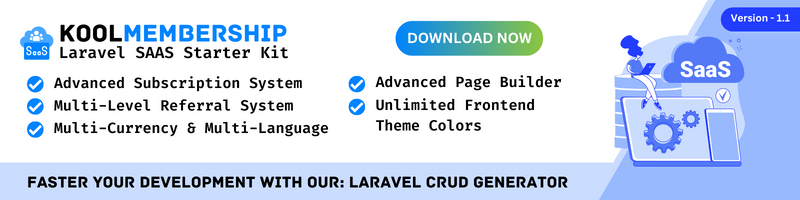Laravel collection to array (`toArray` method) will able us to convert into a plain PHP array for some reason we need to use this in any scenario so that we can access the record through an array.

In this tutorial, I will show you several examples of the Laravel collection to an array.

## Laravel Collection toArray() Example #1:

``````public function index()
{
\$collection = collect(['first_name' => 'Jose', 'last_name' => 'Dela Cruz', 'age' => 20]);

print_r(\$collection->toArray());die;
}

/*
Result.
Array
(
[first_name] => Jose
[last_name] => Dela Cruz
[age] => 20
)

*/``````

As you can see we call the method `toArray()` so that it will return the array we want.

## Laravel Collection toArray() Example #2:

``````public function index()
{
\$collection = collect([
'first_name' => 'Jose',
'last_name' =>
'Dela Cruz',
'age' => 20,
'products' => [
[
'id' => 1,
'name' => 'Product 1',
'description' => 'Product 1 description.',
'price' => 12.99
],
[
'id' => 2,
'name' => 'Product 2',
'description' => 'Product 2 description.',
'price' => 24.99
]
]
]);

print_r(\$collection->toArray());die;
}``````

The above example is with the multi-dimensional array.

## Laravel Collection toArray() Example #3:

``````public function index()
{
\$users = User::all();

print_r(\$users->toArray());die;
}``````

In the above example, we convert the eloquent model result to an array.

``````public function index()
{
\$user = User::find(1);
\$items = \$user->items()->get();

print_r(\$items->toArray());die;
}``````

Another example is if you want to convert the eloquent model result from the relationship to array.